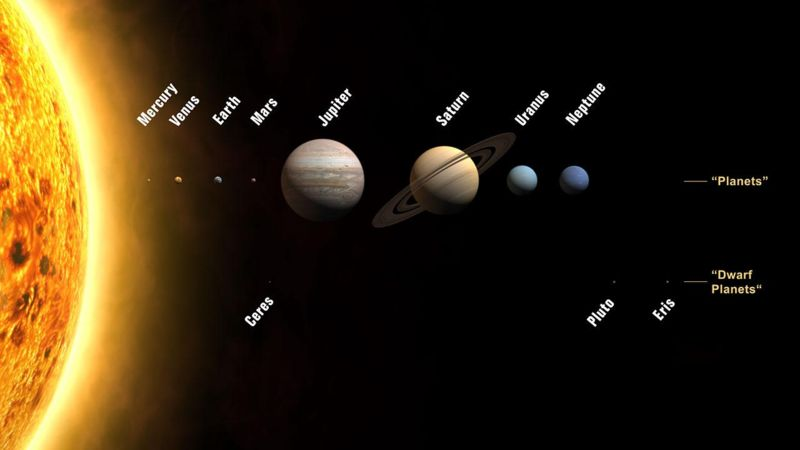The Tilak Hypothesis -

Vedic Age = 5567yearsThe Start of the Tropical Zodiac,
That Celestial circle of 360 degrees,
Is Zero Degree Aries, Vernal Equinox,
The Tropical Zero Point, Sayana Meshadi.

This Zero Point precedes, moving backwards,
One degree, every seventy two years.
( Value given by Cheiro, Count Louis Hamon.}
So Precessional Cycle =72*360= 25920 years,
And this is called the Cosmic Clock.

Orion, Arctic Home in the Vedas,
Is a book by Bala Gangadhar Tilak.
In it he states that the Zero Point,
Was in Head of Orion, Mrigasira,
{Which starts from 53 deg 20 mins,
In the Zodiac of three sixty}.

Now the Tropical Zero Point,
Is at 6 Degrees Pisces,
Which means it has traversed,
Thirty - Six, twenty four degrees !

We have said that the Zero Point moves,
And it has moved 53.20 + 24 =77.3 degrees.
And when we multiply it by 72,
We get the Vedic Age, 77.3*72 = 5567 years.